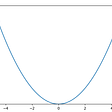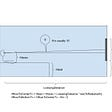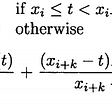# First of all, what is a Norm? In Linear Algebra, a Norm refers to the total length of all the vectors in a space.

There are different ways to measure the magnitude of vectors, here are the most common:

## L0 Norm:

It is actually not a norm. (See the conditions a norm must satisfy here). Corresponds to the total number of nonzero elements in a vector.

For example, the L0 norm of the vectors (0,0) and (0,2) is 1 because there is only one nonzero element.

A good practical example of L0 norm is the one that gives Nishant Shukla, when having two vectors (username and password). If the L0 norm of the vectors is equal to 0, then the login is successful. Otherwise, if the L0 norm is 1, it means that either the username or password is incorrect, but not both. And lastly, if the L0 norm is 2, it means that both username and password are incorrect.

## L1 Norm:

Also known as Manhattan Distance or Taxicab norm. L1 Norm is the sum of the magnitudes of the vectors in a space. It is the most natural way of measure distance between vectors, that is the sum of absolute difference of the components of the vectors. In this norm, all the components of the vector are weighted equally.

Having, for example, the vector X = [3,4]:

The L1 norm is calculated by

As you can see in the graphic, the L1 norm is the distance you have to travel between the origin (0,0) to the destination (3,4), in a way that resembles how a taxicab drives between city blocks to arrive at its destination.

## L2 norm:

Is the most popular norm, also known as the Euclidean norm. It is the shortest distance to go from one point to another.

Using the same example, the L2 norm is calculated by

As you can see in the graphic, L2 norm is the most direct route.

There is one consideration to take with L2 norm, and it is that each component of the vector is squared, and that means that the outliers have more weighting, so it can skew results.

## L-infinity norm:

Gives the largest magnitude among each element of a vector.

Having the vector X= [-6, 4, 2], the L-infinity norm is 6.

In L-infinity norm, only the largest element has any effect. So, for example, if your vector represents the cost of constructing a building, by minimizing L-infinity norm we are reducing the cost of the most expensive building.

I hope you find this article clear and easy to digest, in any other case, feel free to put your question in the comment section or in Twitter. I’ll be happy to clarify any question.

--

--

--

## More from Sara Iris Garcia

Computer science engineer and Machine Learning enthusiast, visit my website https://montjoile.github.io. Follow me on Instagram sara_codes or Twitter @montjoile

Love podcasts or audiobooks? Learn on the go with our new app.

## A Ritual For Making Your Wishes Come True!## Derivation of the quadratic formula## TV mounting height## BSpline Curves — Open-Uniform Knot Vectors## Reinventing Histograms Part 1## Cryptography 101: RSA Algorithm## Mathematical induction: What is it, what’s used for, and how to apply it.## Naive Bayes Classifier deriving from Bayes Theorem## Sara Iris Garcia

Computer science engineer and Machine Learning enthusiast, visit my website https://montjoile.github.io. Follow me on Instagram sara_codes or Twitter @montjoile

## COVID-19: A Poisson Process## Introduction to Explainable Artificial Intelligence in medical imaging## What Statistics Never Told You About Ratings## 3. Hypothesis Testing (z-tests vs t-tests): A Journey to Inner Serenity with Statistics